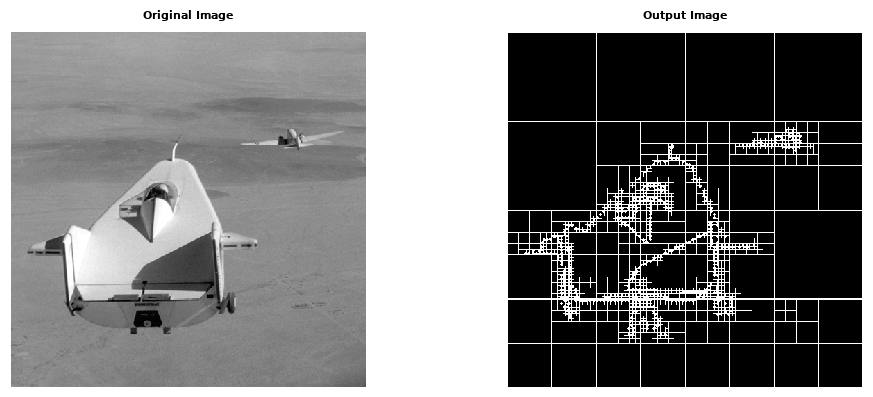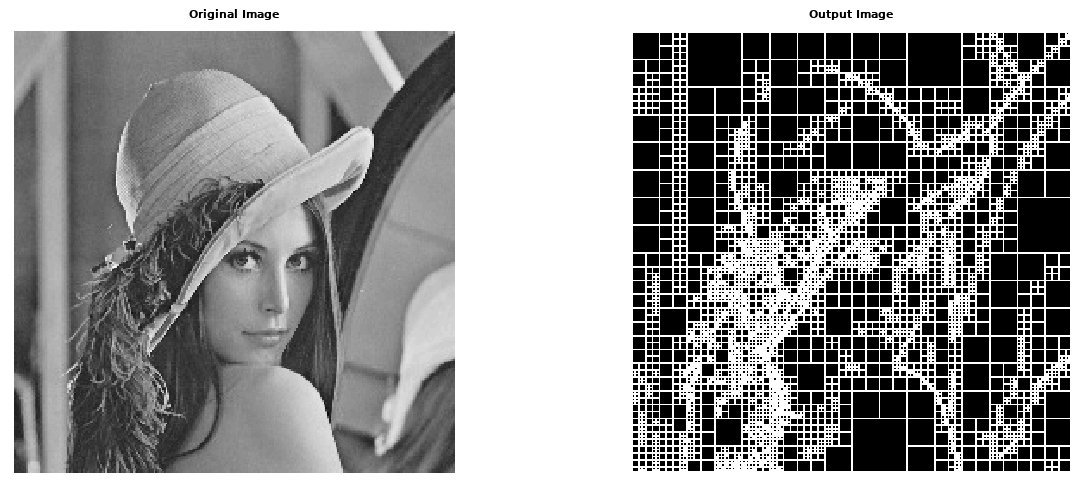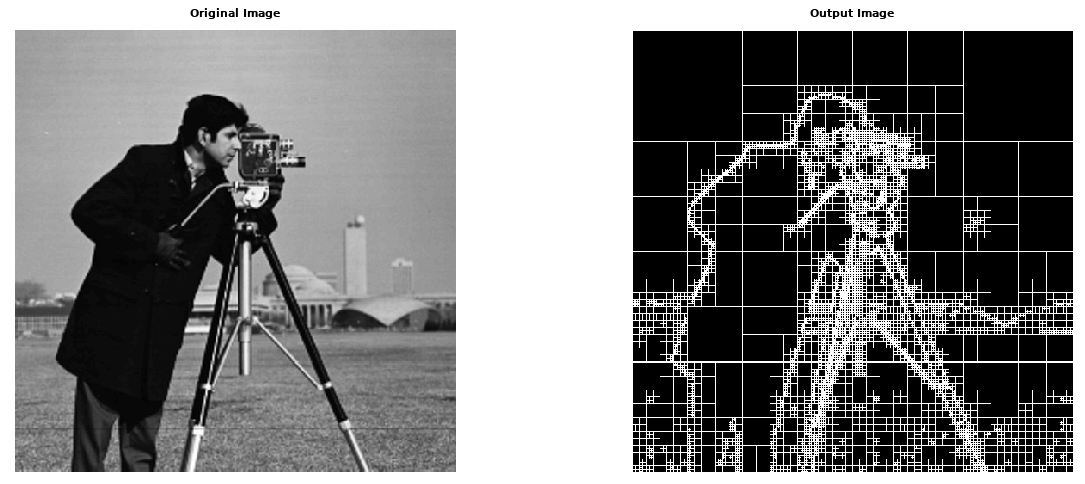# Region Splitting 

## Introduction

Split and merge segmentation is an image processing technique used to segment an image. The image is successively split into quadrants based on a homogeneity criterion and similar regions are merged to create the segmented result. The technique incorporates a quadtree data structure, meaning that there is a parent-child node relationship. The total region is a parent, and each of the four splits is a child.

## Theory

The basic idea of region splitting is to break the image into a set of disjoint regions which are coherent within themselves:

• Initially take the image as a whole to be the area of interest.
• Look at the area of interest and decide if all pixels contained in the region satisfy some similarity constraint.
• If TRUE then the area of interest corresponds to a region in the image.
• If FALSE split the area of interest (usually into four equal sub-areas) and consider each of the sub-areas as the area of interest in turn.
• This process continues until no further splitting occurs. In the worst case this happens when the areas are just one pixel in size.
• This is a divide and conquer or top down method.

If only a splitting schedule is used then the final segmentation would probably contain many neighboring regions that have identical or similar properties. Thus, a merging process is used after each split which compares adjacent regions and merges them if necessary. Algorithms of this nature are called split and merge algorithms. To illustrate the basic principle of these methods let us consider an imaginary image.• Let I denote the whole image shown in (a).
• Not all the pixels in I are similar so the region is split as in (b).
• Assume that all pixels within regions I1, I2 and I3 respectively are similar but those in I4 are not.
• Therefore I4 is split next as in (c).
• Now assume that all pixels within each region are similar with respect to that region, and that after comparing the split regions, regions I43 and I44 are found to be identical.
• These are thus merged together as in (d).

After each split, a test is necessary to determine whether each new region needs further splitting. The criterion for the test is the homogeneity of the region. There are several ways to define homogeneity, some examples are:

• Uniformity - the region is homogeneous if its gray scale levels are constant or within a given threshold.
• Local mean vs global mean - if the mean of a region is greater than the mean of the global image, then the region is homogeneous
• Variance - the gray level variance is defined aswhere r and c are row and column, N is the number of pixels in the region andAn example incorporation would be that the variance of a region be less than a specified value in order to be considered homogeneous.

The splitting results in a partitioned image as shown below to 3 levels.Each level of partitioning can be represented in a tree-like structure.## Algorithm

• Define the criterion to be used for homogeneity
• Split the image into equal size regions
• Calculate homogeneity for each region
• If the region is homogeneous, then merge it with neighbors
• The process is repeated until all regions pass the homogeneity test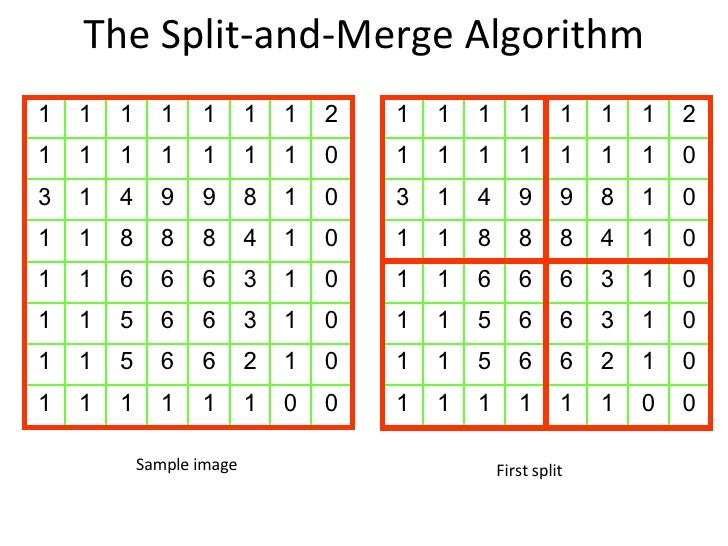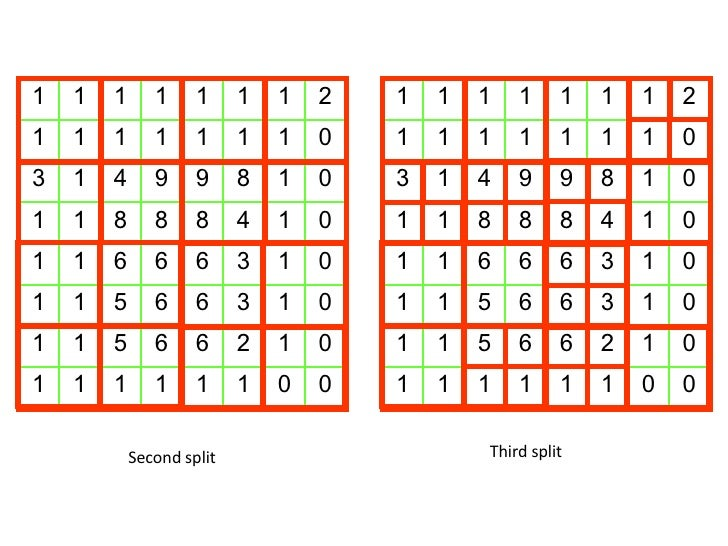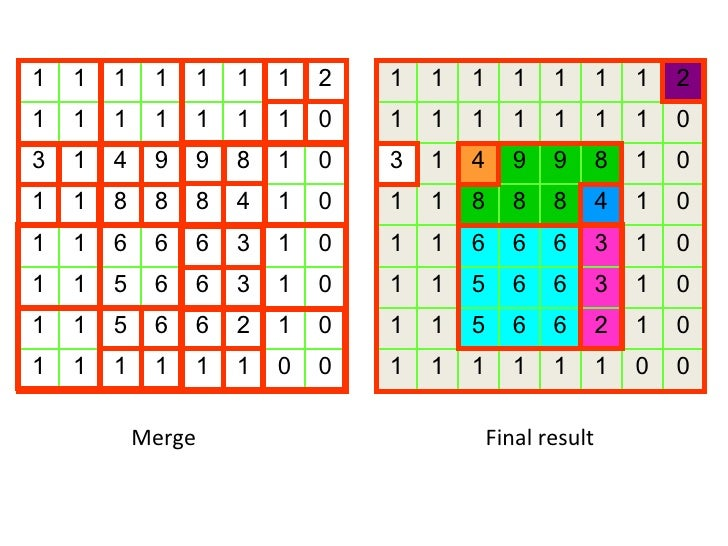## Code

MATLAB function: Quadtree decomposition

```S = qtdecomp(I, threshold, [mindim maxdim])
```

Sample Code:

```I = imread('images/cameraman.jpg');

S = qtdecomp(I, 0.4);
blocks = repmat(uint8(0), size(S));

for dim = [512 256 128 64 32 16 8 4 2 1];
numblocks = length(find(S==dim));
if (numblocks > 0)
values = repmat(uint8(1), [dim dim numblocks]);
values(2:dim,2:dim,:) = 0;
blocks = qtsetblk(blocks,S,dim,values);
end
end

blocks(end, 1:end) = 1;
blocks(1:end, end) = 1;

figure(1);
subplot(1,2,1);
imshow(I);
title('Original Image');

subplot(1,2,2);
imshow(blocks,[]);
title('Output Image');
```

## Output